## Wednesday, August 11, 2021

### TibiaGoals Riddles E11R02

Comment down below!

1.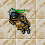Tamarizz - Solidera

35!
Falcon rod = 15
Golden Boots = 10 (each boot 5)
Mage's Cap = 4

Equation would be 15 + (5x4)
15 + 20
35

2.24

Lovely olka

3.oi! I'll go with 35. :)
Thanks and have a great Wednesday!
- Elyrea

4.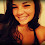55! (Jenny bambina)

5.55! (Jenny bambina)

6.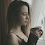Tynusiiaa/Antica
Falcon rod 15, golden boots 10 (so one is 5) and mage's cap 4.

7.35

Shizurie

8.35

Xarkost Eilia

9.10.15+5 x 4 = 80
Vysterion

11.55

Char: Marulinha Chatinha

12.13.35 righo solidera

14.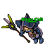You can always check Riddles/Information if your answer was counted as a correct one. If you have a heart next to your nickname in Riddle#2, then it was accepted, if not, it is either a wrong answer or we didn't check it yet.

In this riddle few people provided an incorrect answer already, you still have time till server save to correct it.

15.16.29! /Magiska Malin

17.15 + 5 x 4 = 35

Yeka o/

18.lets try again lol

80!

Magiska Malin

19.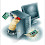is 24 hahaha, Dioyerdj, Celesta

20.35! Character name: Bloth Kalas

21.22.Ops, i would like to change my answer
It's 35 ofc, I didn't notice multiplication sign

Lovely olka

23.The result is 55
Character: Griggi
World: Belobra

24.25.26.27.the answer is 35 because you need to multiply first the 4 x 5 which is 20 then add the 155 that represents the falcon rod. ~Fake Addled

28.35, Lord Neganek

29.35
Mian Stone'arrow

30.15+15=30
10+10 =20
4+4 =8
15+5*4=80.... Bebitha consentida

31.15+15=30
10+10 =20
4+4 =8
15+5*4=80.... Bebitha consentida

32.respuesta es

33.Falcon Rod = 15
Golden Boots = 10
Mage's Cap = 4

So 15 + 5 * 4 = 80

Char: Dyaniixz

34.Falcon Rod = 15
Golden Boots = 10
Mage's Cap = 4

So 15 + 5 * 4 = 80

Char: Dyaniixz

35.15+15 = 30
10+10 = 20
4+4 = 8

15+5*4 = 35

Char: Dark Black Magician

36.37.La respuesta es 80, Madeoz - Menera

38.Falcon rod = 15, pair of golden boots = 10, single golden boot = 5, mage's cap = 4

15+(5*4)=35

39.35 /Norelli

40./Voxarr

41.15 + 5 x 4 = 35
Character: Amy Meow

42.35

Skyelie Hasulhoff <3

43.1. 15 + 15 = 30
2. 10 + 10 = 20
3. 4 + 4 = 8
4. 15 + 5 times 4 = 35

Toxic Hasulhoff

44.45.46.MYO DISTURBED

47.Falcon Rod = 15
Golden Boots = 10
Mage's Cap = 4

15 + 5 * 4 = ?
5 * 4 = 20
15 + 20 = 35

Char: Dyaniixz

48.character name: Pablo Reformed

49.character name: Pablo Reformed

50.character name: Pablo Reformed

51.Name: Archangel Rei
The falcon rod is worth 15 each, the golden boots are worth 10 each and the mage's cap is worth 4 each.
So, we are left with: 15+10x4. Since division and multiplication must be solved first (regardless of the order they appear), we are left with:
15+(10x4)=
15+(40)=
55

52.third times the charm

Magiska Malin

53.This riddle was a tricky one, huh? Correct answer is indeed 35.

54.55.